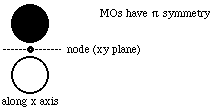Group Theory Solutions: #8

8.* (1994 1 1) Shown below are the one s, three p, and five d atomic orbitals with their phases indicated by shading. Pay special attention to the axes, which are not all the same!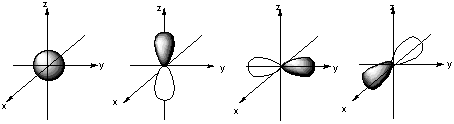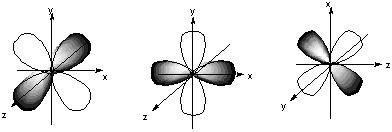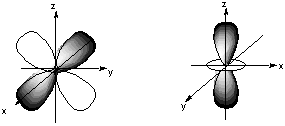A. Classify these nine atomic orbitals by their symmetry under inversion, that is, by their wavefunctions' change in sign when the symmetry operation i is performed. Which of these atomic orbitals are even (do not change sign) and which are odd (do change sign) under inversion?

The s orbital and the 5 d orbitals are even under inversion. The 3 p orbitals are odd under inversion.

B. Consider a py atomic orbital on one nuclear center A and one of the five d atomic orbitals on another nuclear center B. Let the nuclear centers A and B be placed on the x axis with A on the left and B on the right. As A and B approach, molecular orbitals can be formed from the two atomic orbitals if these two atomic orbitals have the same symmetry with respect tot he internuclear axis. With which atomic d orbital on B is there a nonzero (noncancelling) overlap with the atomic py orbital on A?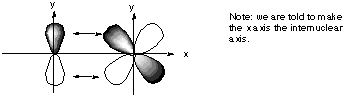All d orbitals except the dxy have the wrong spatial orientation for interaction with the py orbital along an internuclear axis defined as the x axis.

C. Draw pictures of the bonding and anitbonding molecular orbitals that result from this linear combination of atomic orbitals.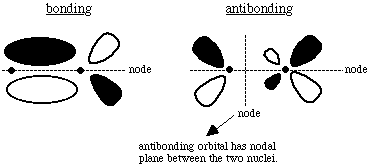D. Classify the resulting molecular orbital as to its symmetry with respect to the internuclear axis.

If we look along the internuclear axis of either the bonding or the antibonding MO, we notice a nodal plane containing x: